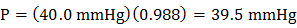### Sample Problem

At 19 °C, the vapor pressure of pure ethanol (molar mass = 46.1 g) is 40.0 mmHg. If 10.0 gram of sodium hydroxide (molar mass = 40.0 g/mol) was dissolved in 922 g of ethanol, what is the vapor pressure of the resulting solution? Assume the solution is ideal. mmHg

#### Solution